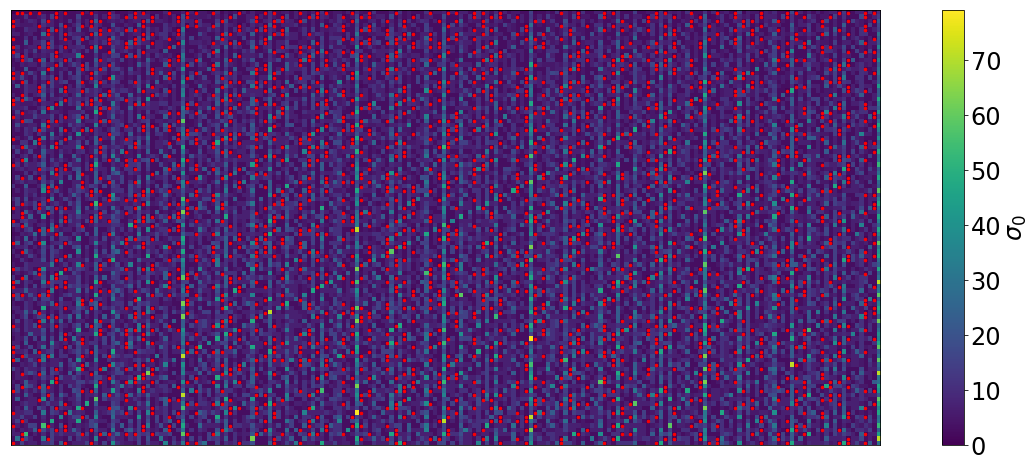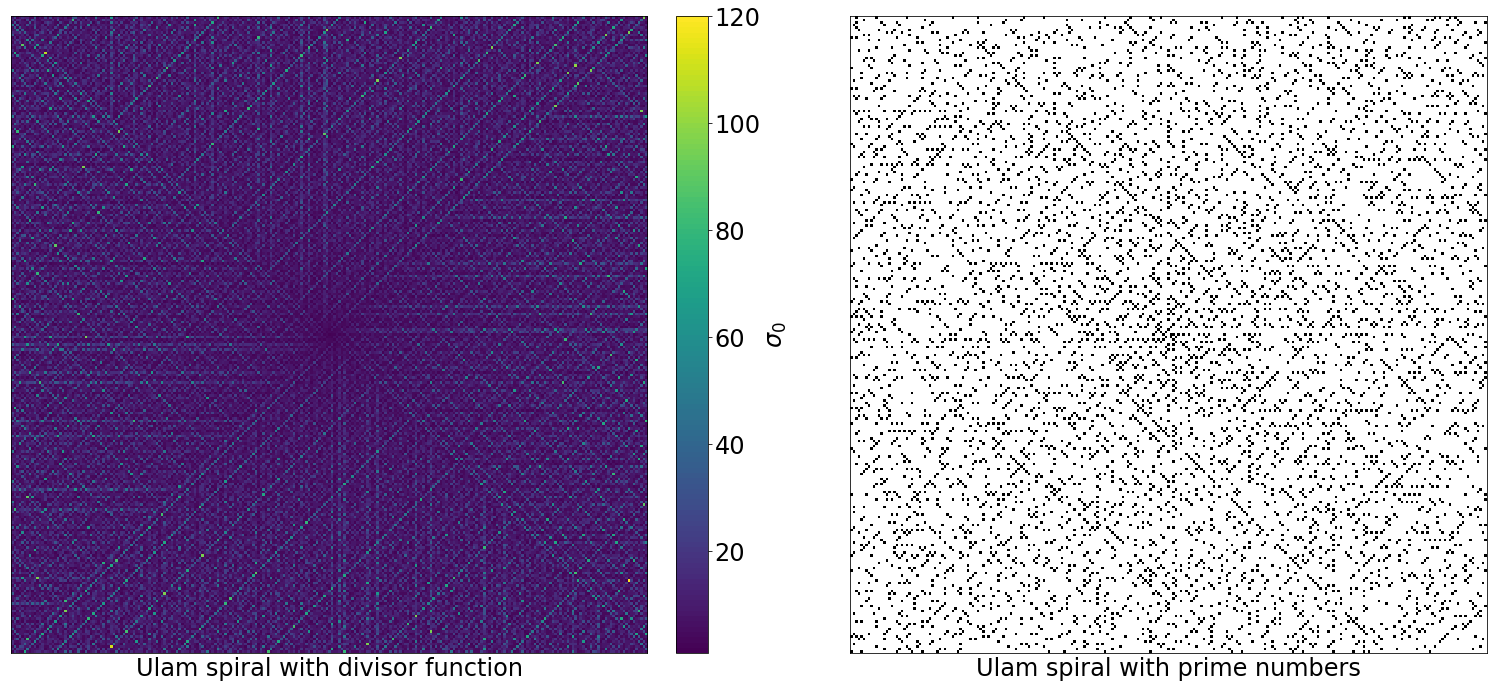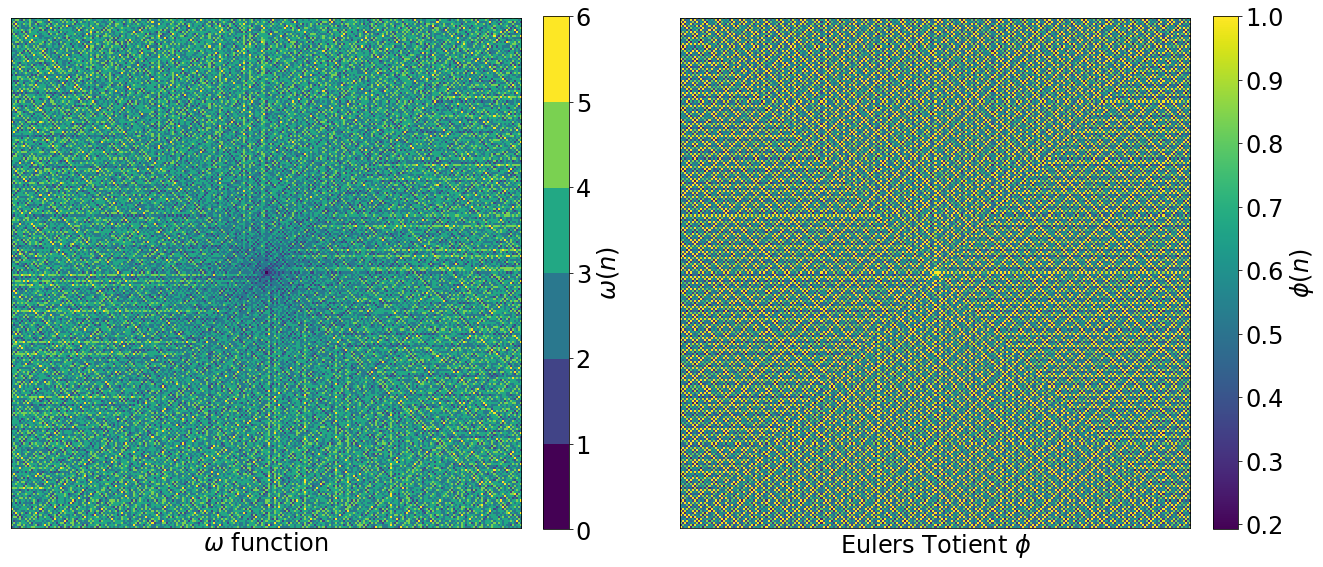# Primes Sonification

## How does prime numbers distribution sounds like?

Ansatz
, in 17 April 2017
primesound

## A bit of number theory¶

Are prime number distributed randomly? Probably not.

One way to see prime numbers is as special cases of some functions. For example, the divisor function $\sigma_0(n)$, is the function that counts how many divisor a number $n$ has. A number is prime if the function equals two (one and itself). The following image contains the first 20000 divisor function values for natural numbers from top to bottom and left to right. The value two (prime numbers) is highlighted.Although some patter arise, this depends on the number of columns. To avoid a privileged ordering, the Ulam spiral is employed.

$$17\; 16\; 15\; 14\; 13 \\ 18\;\; 5\;\; 4\;\; 3\;\; 12\\ 19\;\; 6\;\; 1\;\; 2\;\; 11\\ 20\;\; 7\;\; 8\;\; 9\;\; 10\\ 21\; 22\; 23\; 24\; 25$$

Let see the divisor function and the prime numbers in this spiral setup. All following spirals go from 1 to 63001.The Ulam spiral shows something beautiful and complex is going on there. Another related magnitude to describe prime numbers is $\omega(n)$, the number of distinct prime factors of $n$. So for a prime number, the value is 1.

Another pattern arise when plotting $\phi(n)$, Euler's totient function. For given $n$, this function counts the quantity of coprimes with $n$. A prime number has $n$ - 1 comprimes, every number beneath them minus their self. The figure show a normalized $\tilde{\phi}(n)=(\phi(n)+1)/n$, so prime numbers have a value of one.Lets consider one more function. The one that, for a given $n$, counts the distance to the next prime number, so in a sense it represents the prime numbers gap. This gap value is bounded (next prime bound).